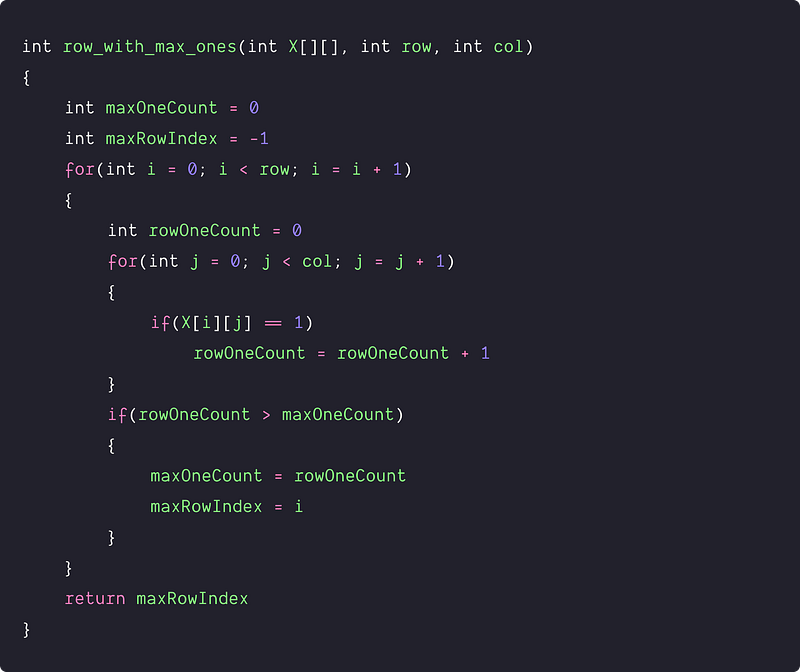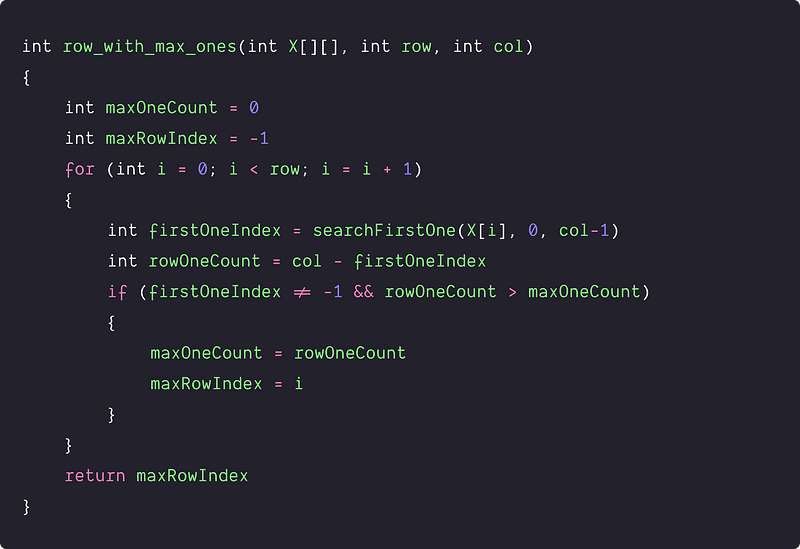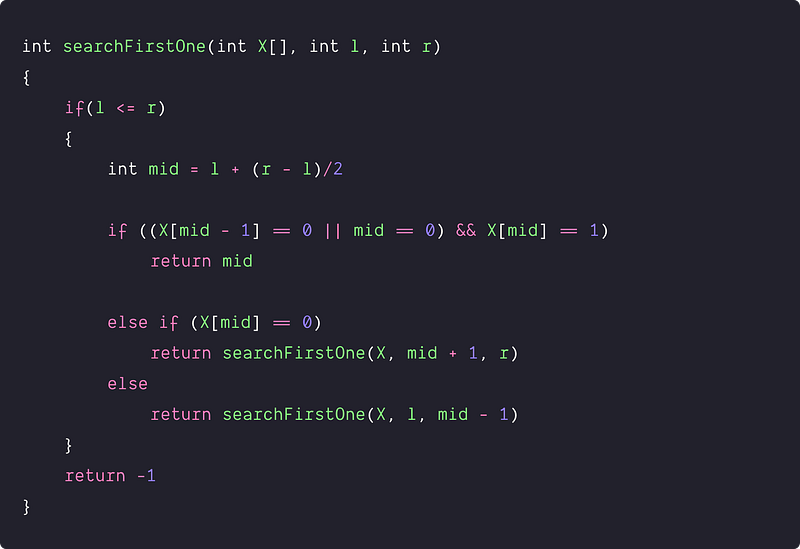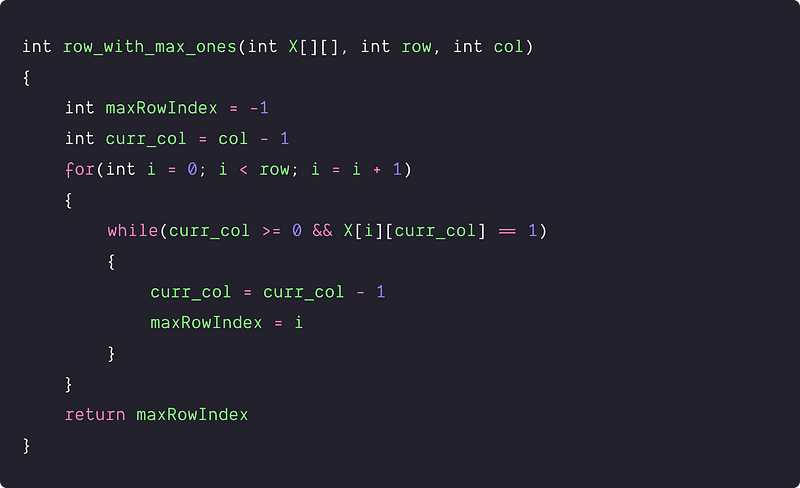# Find the row with the maximum number of 1s

Difficulty: Medium, Asked-in: Amazon, Microsoft, Paytm

Key takeaway: This is an excellent matrix problem that can be solved in linear time complexity. We are using the sorted order property and nested loops to improve the solution over the binary search approach.

### Let’s understand the problem

Given a boolean 2D array of size m x n, where each row is sorted. Find the row with the maximum number of 1s.

Examples

``````Input: X[][]
0 1 1 1
1 1 1 1 <- row with max number of 1's
0 0 1 1
0 0 0 0

Output: 1 (0-based indexing)

Input: X[][]
0 0 1 1
0 0 0 1
0 1 1 1 <- row with max number of 1's
0 0 0 1

Output: 2
``````

### Discussed solution approaches

• A brute force solution using row-wise traversal
• Using row-wise binary search
• An efficient solution using two nested loops

### A brute force solution using row-wise linear search

Solution Idea

A straightforward method is to do a row-wise linear search, count the number of 1s in each row and track the row-index with a max 1 count. To implement this, we use two nested loops and three variables:

• rowOneCount to track the count of 1's in each row.
• maxOneCount to track the count of the max number of 1's.
• maxRowIndex to track the row-index with max number of 1's.

Solution PseudocodeTime and space complexity analysis

We are traversing each matrix element using nested loops and doing O(1) operation at each iteration. So time Complexity = n*m*O(1) = O(nm). We are using constant extra variables, so space complexity = O(1).

### Using the idea of binary search

Solution Idea

We already know that each row is sorted. Can we use this information to improve the time complexity? Let's think!

• For each row, instead of using linear search, we can apply binary search to find the first instance of 1, where the binary search will return the index of the first occurrence.
• Now we can easily calculate the number of 1s in the row, which is equal to the total number of columns minus the index of the first 1.
• During this process, we also update the count of the max number of 1s and row index with max 1's.

Solution Steps

We declare four variables to track the count of 1's in a row(rowOneCount), overall max count of 1's (maxOneCount), index of the first occurrence of 1 (firstOneIndex), and row-index with max number of 1's (maxRowIndex). For accessing each row, we run a loop from i = 0 to row - 1:

• For each row i, we start by searching the first occurrence of 1 using the binary search i.e. firstOneIndex = searchFirstOne(X[], 0, col - 1). We are passing 0 as the left parameter and col -1 as the right parameter because the total number of elements in each row is equal to the total number of columns.
• Now we calculate the total number of 1's in each row i.e rowOneCount = col - firstOneIndex
• if(firstOneIndex != -1 && rowOneCount > maxOneCount), we update the value of maxOneCount with rowOneCount and maxRowIndex with i.

``````if (firstOneIndex != -1 && rowOneCount > maxOneCount)
{
maxOneCount = rowOneCount
maxRowIndex = i
}
``````
• By end of the loop, we return the value stored in maxRowIndex.

Solution PseudocodeSearching the first occurrence of 1: searchFirstOne(X[], l, r)

As mentioned above, we can apply the binary search to find the first occurrence of the one. For this, we are using recursive binary search where the base case occurs when l > r. So if(l <=r), we execute the following steps:

• Calculate the middle index i.e. mid = l + (r - l)/2
• If the value at mid is 1 and the previous value at mid - 1 is 0, it means, the first 1 is present at the mid index. There is another corner case when the first one is present at the first position: if mid = 0 and X[mid] == 1. In both situations, we return mid-index as the first occurrence.

``````if ((X[mid - 1] == 0 || mid == 0) && X[mid] == 1)
return mid
``````
• If(X[mid] == 0), all the left half values will be zero, and the first 1 may be present somewhere in the right half. So we search the occurrence of the first 1 in the right half using the same function recursively with mid + 1 and r as the left and right end.
• Otherwise, if both the above conditions are false, then the mid index is at some 1, not the first occurrence. In other words, the first one will be present somewhere in the left half. So we need to search the occurrence of the first 1 in the left half using the same function recursively with l and mid - 1 as the left and right end.

``````else if (X[mid] == 0)
return searchFirstOne(X, mid + 1, r)
else
return searchFirstOne(X, l, mid - 1)
``````
• When recursion reaches the base case, it means we didn't find any 1 in the array. We return -1.

Pseudocode: Searching the first occurrence of 1Time and space complexity analysis

Time Complexity = number of rows * time complexity of binary search to find first 1 in each row = n * O(logm) = O(nlogm), where n is the number of rows, and m is the number of columns.

We are using recursive binary search, so space complexity depends on the size of the call stack which is equal to the height of the recursion tree. Space Complexity = O(logn) (Think!)

### An efficient approach using two nested loops

Solution Idea

Can we improve the time complexity further? Think! Here are two observations from the given input matrix: 1) Each row is sorted, so all the 1's in any row will be lined up to the right end. 2) For the first 1 in any row, if there is a 1 in the same column in the next row, then the count of 1's in row <= count of 1's in the next row. The same idea applies to all the consecutive i and i + 1 rows.

So here is an exciting solution idea:

• Start from the top-right corner of the matrix and continue moving left until we find 0. During the process, we are updating the value of the max row index.
• Now, continue moving downward in that column until we came across 1 in some row i. In this case, all the intermediate rows from 1 to (i-1) have numbers of 1's less than the first row.
• Again, in row i, continue moving left until we find 0 and update the value of max row-index.
• We will continue the above process until we reach the last row.

Solution Steps

1. We initialize the variable maxRowIndex to track the row index with max 1 and curr_col to track the current column.
2. Now we run a loop from i = 0 to row - 1:

• If(currcol >= 0 && X[i][currcol] == 1), we continue moving left from the top right corner until we find 0. We also keep updating the value of the maxRowIndex.
``````while(curr_col >= 0 && X[i][curr_col] == 1)
{
curr_col = curr_col - 1
maxRowIndex = i
}
``````
• When the above condition is false, we continue moving downward in each column by incrementing the value of i until we came across 1 in some row.
• The above process will continue till i = row.
• By end of the loop, we return the value stored in the maxRowIndex.

Solution PseudocodeTime and space complexity analysis

There are two nested loops in the code, and time complexity looks O(mn), but this is not the correct analysis. If we observe closely, we traverse each row and column once, i.e outer loop traverses each row, and the inner loop traverses each column (Think!).

Let's think from another perspective: when we are traversing any row from left to right, then all the columns in that path will be traversed once. Similarly, when we travel any column from top to down, all the rows in that path will be traversed once. (Think!)

• Total loop iteration = total number of rows + total number of columns = m + n.
• At each loop iteration, we are doing an O(1) operation.
• Time Complexity = Total loop iteration * O(1) = (m + n) * O(1) = O(m + n)

We are using a constant number of variables, so space complexity = O(1)

### Critical ideas to think!

• What is the worst and best case scenario of the efficient approach?
• Why are we starting from the top right corner? can we solve the problem from a different starting point?
• Can we implement the 2nd solution using the iterative binary search?
• Why are we moving in the same row if the current cell has a value of 1?

### Comparisons of time and space complexities

• Using row-wise traversal: Time = O(nm), Space = O(1)
• Using binary search: Time = O(nlogm), Space = O(logn)
• Using nested loops: Time = O(m + n), Space = O(1)

### Matrix-based problems for practice

Enjoy learning, Enjoy coding, Enjoy algorithms!

Get well-designed application and interview centirc content on ds-algorithms, machine learning, system design and oops. Content will be delivered weekly.